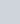Obtain the resonant frequency and Q-factor of a series LCR circuit with
Question:

Obtain the resonant frequency and Q-factor of a series LCR circuit with = 3.0 H, = 27 μF, and = 7.4 Ω. It is desired to improve the sharpness of the resonance of the circuit by reducing its ‘full width at half maximum’ by a factor of 2. Suggest a suitable way.

Solution:

Inductance, L = 3.0 H

Capacitance, C = 27 μF = 27 × 10−6 F

Resistance, R = 7.4 Ω

At resonance, angular frequency of the source for the given LCR series circuit is given as:

$\omega_{r}=\frac{1}{\sqrt{L C}}$

$=\frac{1}{\sqrt{3 \times 27 \times 10^{-6}}}=\frac{10^{3}}{9}=111.11 \mathrm{rad} \mathrm{s}^{-1}$

Q-factor of the series:

$Q=\frac{\omega_{r} L}{R}$

$=\frac{111.11 \times 3}{7.4}=45.0446$

To improve the sharpness of the resonance by reducing its ‘full width at half maximum’ by a factor of 2 without changing, we need to reduce R to half i.e.,

Resistance $=\frac{R}{2}=\frac{7.4}{2}=3.7 \Omega$